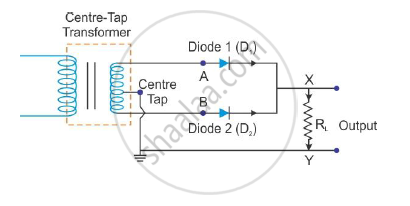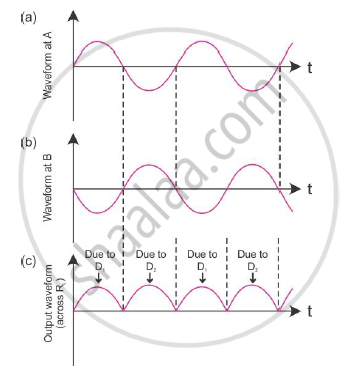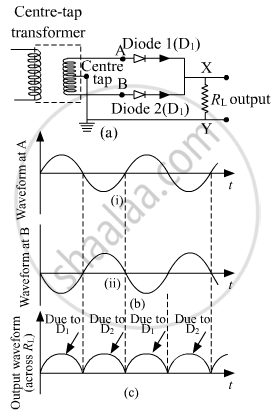# Draw the Circuit Diagram of a Full Wave Rectifier Along with the Input and Output Waveforms - Physics (Theory)

Draw the circuit diagram of a full wave rectifier along with the input and output waveforms

Draw the circuit diagram of a full wave rectifier and state how it works.

Draw the circuit diagram of a full wave rectifier. Explain its working showing its input and output waveforms.

#### Solution 1

Full wave rectifier:Input and output waveforms:During positive half cycle of the A.C. input point A is + ve w.r.t. point C, (and point B is – ve w.r.t. point C), therefore the diode D1 is forward biased and D2 is reverse biased.
The current flows through diode D1, RL and upper half winding (AC).
During every negative half cycle of A.C. input, point B is + ve w.r.t point C (and point A is – ve w.r.t. point
C), therefore diode D2 is forward biased and diode D1 is reverse biased.
Current flows through RL, from point M to point N (unidirectional).

#### Solution 2

The circuit diagram and the associated waveforms are shown below:(a) Full-wave rectifier circuit
(b) Input waveforms are given to the diode D1 at A and to the diode D2 at B
(c) Output waveform across the load RL connected in the full-wave rectifier circuit

A Full Wave Rectifier is a circuit, which converts an ac voltage into a pulsating dc voltage using both half cycles of the applied ac voltage. It uses two diodes of which one conducts during one-half cycle while the other conducts during the other half cycle of the applied ac voltage.
During the positive half cycle of the input voltage, diode D1 becomes forward biased and D2 becomes reverse biased. Hence D1 conducts and D2 remains OFF. The load current flows through Dand the voltage drop across RL will be equal to the input voltage.
During the negative half cycle of the input voltage, diode D1 becomes reverse biased and D2 becomes forward biased. Hence D1 remains OFF and D2 conducts. The load current flows through Dand the voltage drop across RL will be equal to the input voltage.

Concept: Diode as a Rectifier
Is there an error in this question or solution?
2015-2016 (March) All India Set 3 N

Share